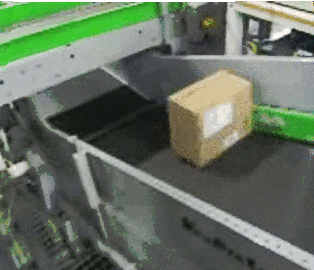Chapter 10

## Tinkering with integers and basic math in SmartPy

### Intro:

Let us quickly understand how integers are handled under the hood in SmartPy!

In the later half of the chapter, you will also learn to add movement ability to our `Cryptobot`!## Study Time:

### Integer:

The type of integer values i.e -42, 4, 10000.

Most common use cases of integers are represented by the following types:

1. Non-negative integers: eg: 4 is represented by `sp.TNat`
2. Signed integers (i.e integers that can hold negative values): eg: -42 is represented by `sp.TInt`
``````
## x_coordinate is a variable that
## stores the current x coordinate of your Cryptobot.
## Range between [-10, 10].
## Useful when you want to move your Cryptobot horizontally.
self.init(coordinate_x = 0)

## y_coordinate is a variable
## that stores the current y coordinate of your Cryptobot.
## Range between [1, 5].
## Useful when you want to move your Cryptobot vertically.
self.init(coordinate_y = 1)

## Move 4 steps backward.
self.data.coordinate_x -= 4

## You can also explicitly give type by doing the following:
self.data.coordinate_x = sp.int(-2)
self.data.coordinate_y = sp.nat(3)``````

Notes:

1. You will rarely be setting types for integer values in your code.
2. There is a third type as well called `sp.TIntOrNat` whose type is undetermined between `sp.TInt` or `sp.TNat`. This is an advanced concept which will be taught in later chapters.
3. You can read more in depth about how SmartPy handles integers here.

## #buidl-ing time:

#### New feature request:

Our current Cryptobot lacks the ability to move! It is basically like this package at the moment:How do you imagine a Cryptobot to hide and seek aliens without it being able to move?

#### Step by step walkthrough:

1. Let’s add `coordinate_x`, `coordinate_y` state variables to act as the initial coordinate of our `Cryptobot`.

• Initialize `coordinate_x` with `sp.int(0)` as we know coordinate x can range from [-10, 10] i.e coordinate x can hold signed integers.

• Initialize `coordinate_y` with `sp.nat(0)` as we know coordinate y can range from [0, 5] i.e coordinate y can only hold non-negative integers.

2. Then add two entry point functions `move_horizontally` and `move_vertically` which will update the coordinates of our Cryptobot.

• Both these functions take an argument `update_to` which is used to update the coordinate’s values.

We have left this exercise a little vague so that you can practice working with state variables and entry point functions. Check out Chapter 5 and Chapter 7 for a quick refresher.

#### Testing our code:

Let us test our movement ability by moving it forward by 2 and vertically by 1:

1. Call `move_horizontally` and pass the value `2`
2. Call `move_vertically` and pass the value `1`

When you’re finished:

1. Click on “Check” to check your submission with the correct answer.
2. Click on “Show Answer” in case you get stuck.

You can also tinker around with the final code on SmartPy’s online IDE. Try running the test by clicking on the ▶️ button!

Contract.py